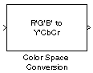Documentation

Color Space Conversion

Convert color information between color spaces

• Library:
• Computer Vision Toolbox / ConversionsDescription

The Color Space Conversion block converts color information between color spaces. Use the Conversion parameter to specify the color spaces you are converting between.

• R'G'B' to Y'CbCr

• Y'CbCr to R'G'B'

• R'G'B' to intensity

• R'G'B' to HSV

• HSV to R'G'B'

• sR'G'B' to XYZ

• XYZ to sR'G'B'

• sR'G'B' to L*a*b*

• L*a*b* to sR'G'B'

Ports

Input

expand all

Input color information, specified as an M-by-N-by-P color matrix of P color planes.

Data Types: single | double | int8 | uint8

R plane of the RGB color information, specified as an M-by-N matrix.

Data Types: single | double | int8 | uint8

G plane of the RGB color information, specified as an M-by-N matrix.

Data Types: single | double | int8 | uint8

B plane of the RGB color information, specified as an M-by-N matrix.

Data Types: single | double | int8 | uint8

Luma signal component, specified as an M-by-N matrix.

Data Types: single | double | int8 | uint8

Chrominance component, specified as an M-by-N matrix.

Data Types: single | double | int8 | uint8

Chrominance component, specified as an M-by-N matrix.

Data Types: single | double | int8 | uint8

Intensity values specified as an M-by-N matrix.

Data Types: single | double | int8 | uint8

Hue component, specified as an M-by-N matrix.

Data Types: single | double

Saturation component, specified as an M-by-N matrix.

Data Types: single | double

Brightness component, specified as an M-by-N matrix.

Data Types: single | double

X component, specified as an M-by-N matrix.

Data Types: single | double

Y component, specified as an M-by-N matrix.

Data Types: single | double

Z component, specified as an M-by-N matrix.

Data Types: single | double

Luminance component, specified as an M-by-N matrix.

Data Types: single | double

a* component, specified as an M-by-N matrix.

Data Types: single | double

b* component, specified as an M-by-N matrix.

Data Types: single | double

Output

expand all

Input color information, specified as an M-by-N-by-P color matrix of P color planes.

• If the input is uint8, then Y'CbCr is uint8, where Y is in the range [16 235], and Cb and Cr are in the range [16 240].

• If the input is a double, then Y' is in the range [16/255 235/255] and Cb and Cr are in the range [16/255 240/255].

Data Types: single | double | int8 | uint8

R plane of the RGB color information, specified as an M-by-N matrix.

Data Types: single | double | int8 | uint8

G plane of the RGB color information, specified as an M-by-N matrix.

Data Types: single | double | int8 | uint8

B plane of the RGB color information, specified as an M-by-N matrix.

Data Types: single | double | int8 | uint8

Luma signal component, specified as an M-by-N matrix.

Data Types: single | double | int8 | uint8

Chrominance component, specified as an M-by-N matrix.

Data Types: single | double | int8 | uint8

Chrominance component, specified as an M-by-N matrix.

Data Types: single | double | int8 | uint8

Intensity values specified as an M-by-N matrix.

Data Types: single | double | int8 | uint8

Hue component, specified as an M-by-N matrix.

Data Types: single | double

Saturation component, specified as an M-by-N matrix.

Data Types: single | double

Brightness component, specified as an M-by-N matrix.

Data Types: single | double

X component, specified as an M-by-N matrix.

Data Types: single | double

Y component, specified as an M-by-N matrix.

Data Types: single | double

Z component, specified as an M-by-N matrix.

Data Types: single | double

Luminance component, specified as an M-by-N matrix.

Data Types: single | double

a* component, specified as an M-by-N matrix.

Data Types: single | double

b* component, specified as an M-by-N matrix.

Data Types: single | double

Parameters

expand all

Specify the conversion color spaces as one of the following:

• R'G'B' to Y'CbCr

• Y'CbCr to R'G'B'

• R'G'B' to intensity

• R'G'B' to HSV

• HSV to R'G'B'

• sR'G'B' to XYZ

• XYZ to sR'G'B'

• sR'G'B' to L*a*b*

• L*a*b* to sR'G'B'

Specify the standard to convert your values between the R'G'B' and Y'CbCr color spaces as either Rec. 601 (SDTV) or Rec. 709 (HDTV).

Dependencies

This parameter is visible when you set the Conversion to R'G'B' to Y'CbCr or Y'CbCr to R'G'B'.

Specify the scanning standard to convert between the R'G'B' and Y'CbCr color spaces as either 1125/60/2:1 or 1250/50/2:1.

Dependencies

This parameter is visible when you set the Use conversion specified by parameter to Rec. 709 (HDTV).

Specify the reference white point.

Dependencies

This parameter is visible when you set the Conversion parameter to sR'G'B' to L*a*b* or L*a*b* to sR'G'B'.

Specify how to input and output a color signal. If you select One multidimensional signal, the block accepts an M-by-N-by-P color signal, where P is the number of color planes, at one port. If you select Separate color signals, additional ports appear on the block. Each port accepts one M-by-N plane of color signal stream.

Note

The prime notation indicates that the signals are gamma corrected.

Block Characteristics

 Data Types Boolean | double | integer | single Multidimensional Signals yes Variable-Size Signals yes

Algorithms

expand all

 Poynton, Charles A. A Technical Introduction to Digital Video. New York: John Wiley & Sons, 1996.

 Recommendation ITU-R BT.601-5, Studio Encoding Parameters of Digital Television for Standard 4:3 and Wide Screen 16:9 Aspect Ratios.

 Recommendation ITU-R BT.709-5. Parameter values for the HDTV standards for production and international programme exchange.

 Stokes, Michael, Matthew Anderson, Srinivasan Chandrasekar, and Ricardo Motta, “A Standard Default Color Space for the Internet - sRGB.” November 5, 1996.

 Berns, Roy S. Principles of Color Technology, 3rd ed. New York: John Wiley & Sons, 2000.